Courses

# Test: Polynomials (Medium)

## 20 Questions MCQ Test | Test: Polynomials (Medium)

Description
This mock test of Test: Polynomials (Medium) for Class 10 helps you for every Class 10 entrance exam. This contains 20 Multiple Choice Questions for Class 10 Test: Polynomials (Medium) (mcq) to study with solutions a complete question bank. The solved questions answers in this Test: Polynomials (Medium) quiz give you a good mix of easy questions and tough questions. Class 10 students definitely take this Test: Polynomials (Medium) exercise for a better result in the exam. You can find other Test: Polynomials (Medium) extra questions, long questions & short questions for Class 10 on EduRev as well by searching above.
QUESTION: 1

### Find the zeros of a quadratic polynomial √3x2 - 8x + 4√3.

Solution: Given quadratic equation = √3x² - 8x + 4√3 = 0

We should factorize the equation first. =√3x² - 6x - 2x + 4√3 = 0

= √3x(x - 2√3) - 2(x - 2√3) = 0

= (√3x - 2) (x - 2√3) = 0

= (√3x - 2) = 0, (x - 2√3) = 0

= x = 2 / √3 , x = 2√3.

QUESTION: 2

### Write the degree of the given polynomial: 2p - √7.

Solution: The highest power of the variable in a polynomial in one variable is called the degree of the polynomial.

QUESTION: 3

### Find the degree of the polynomial 5t + √7.

Solution: The degree is the highest power of the variable in the polynomial.

QUESTION: 4

Find the smallest solution in positive integers of x2 - 14y2 = 1.

Solution: x2 − 1 = 14y2

Now checking by putting y = 1, 2, 3... until we get solution

y = 1; => x2 =15 not possible

y = 2; => x2 = 57 not possible

y = 3; => x2 = 127 not possible

y = 4; => x2 = 225 => x = 15

Hence, the smallest solution is (x, y) = (15, 4).

QUESTION: 5

Find the zeroes of the quadratic polynomial from the graph.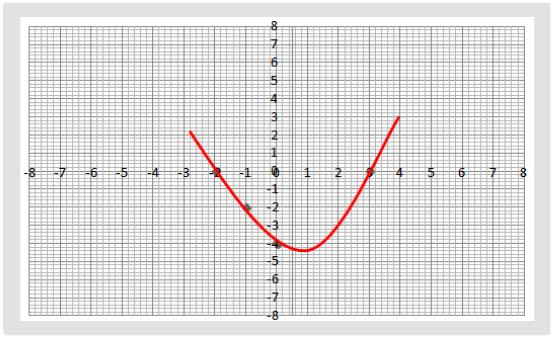Solution: Given polynomial graph cuts the x-axis at 2 points. So, it must have two roots. Here the x-coordinates of (-2, 0) and (3, 0) are the two zeroes of the quadratic polynomial.

QUESTION: 6

If one zero of polynomial (k2 + 16) x2 + 16x + 8k is reciprocal of the other, them k is equal to

Solution:
QUESTION: 7

The graph of f(x) is shown below. The number of zeroes of f(x) are: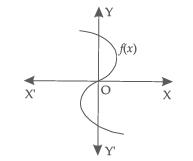Solution:
QUESTION: 8

The graph of a quadratic polynomial p(x) = ax2 + bx + c is a parabola, opening downwards if

Solution:
QUESTION: 9

Sum of the zeroes of the polynomial p(x) = - 3x2 + a is

Solution:
QUESTION: 10

The sum and product of zeroes of a quadratic polynomial are - 1 and - 6 respectively. The quadratic polynomial is given by

Solution: Quadratic equation given sum and product of zeros is,

p(x) = x² - x (sum of zeros) + product of zeros

p(x) = x² - x (-1) + (-6)

p(x) = x² + x - 6

QUESTION: 11

Number of zeroes of a polynomial of degree n is

Solution: A polynomial of n degree can have n zeros. For example, a quadratic equation ax2 + bx + c = 0 can have 2 zeros, as the highest power of x is 2 or as the degree is 2. ax3 + bx2 + cx + d = 0, a cubic equation can have 3 zeros, as the highest power of x is 3 or as the degree is 3.

QUESTION: 12

Number of quadratic polynomials having - 2 and - 5 as their two zeroes is

Solution: Let p (x) = ax2 + bx + c be the required polynomial whose zeroes are -2 and 5.

Hence, the required number of polynomials are infinite i.e., more than 3.

QUESTION: 13

Which of the following is not a graph of a quadratic polynomial?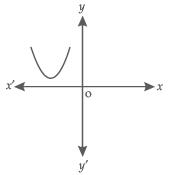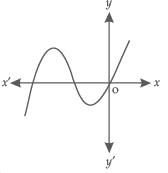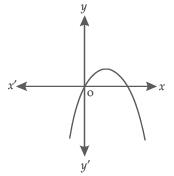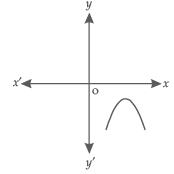Solution:
QUESTION: 14

If one zero of the quadratic polynomial 39 y2 - (2k + 1)y - 22 is negative of the other, then the value of k is

Solution:
QUESTION: 15

If α and β are zeros of a quadratic polynomial such that α + β = 12 and α - β = 6. Them, the family of quadratic polynomials having α, β as its zeroes is given by

Solution:
QUESTION: 16

If α, β, γ are the zeroes of the polynomial p(x) = x3 + 6x2 + cx + d, such that α + β = 2, then the value of γ is

Solution: Sum of roots = −b / a​ = −6

⇒ α + β + γ = −6

⇒ 2 + γ = −6

⇒ γ = −8

QUESTION: 17

If the zeroes of the quadratic polynomial x2 + (a +1) x + b are 2 and - 3, then

Solution: Thus, 2 + (−3) = 1 − (a + 1)

=> 1 (a + 1) = 1

=> a + 1 = 1

=> a = 0

Also, 2 × (−3) = b = -6

QUESTION: 18

When x4 + x3 - 2x2 + x + 1 is divided by x - 1, them the remainder is

Solution:
QUESTION: 19

If α and β are the zeroes of the quadratic polynomial p(x) = x2 + 2x - k such that α2 + β2 = 34, then the value of k is

Solution: α + β = -b / a

α + β = -2

α x β = c / a

α x β = -k

Now, α2 + β2 = 34

using identity we get

(α + β)2 - 2α x β

Now putting up the values

(-2)2 - 2 x -k = 34

4 + 2k = 34

2k = 30

k = 15.

QUESTION: 20

If the sum of products of zeroes taken, two at a time of polynomial p(x) = x3 - 5x2 + kx + 8 is 2, then the value of k is

Solution: